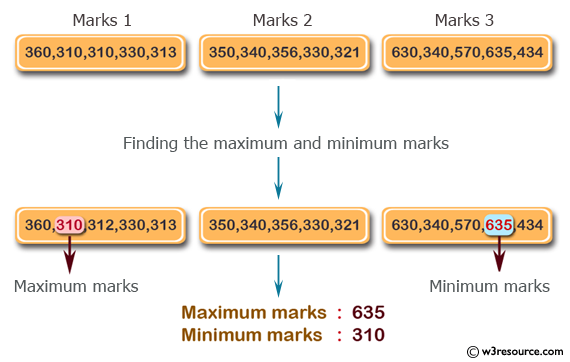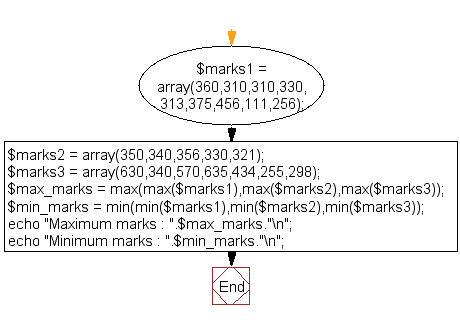﻿ PHP Math Exercise: Find the maximum and minimum marks from the specified set of arrays - w3resource# PHP Math Exercises: Find the maximum and minimum marks from the set of arrays

## PHP math: Exercise-1 with Solution

Write a PHP script to find the maximum and minimum marks from the following set of arrays.

Sample arrays :
\$marks1 = array(360,310,310,330,313,375,456,111,256);
\$marks2 = array(350,340,356,330,321);
\$marks3 = array(630,340,570,635,434,255,298);

Pictorial Presentation:Sample Solution:

PHP Code:

``````<?php
\$marks1 = array(360,310,310,330,313,375,456,111,256);
\$marks2 = array(350,340,356,330,321);
\$marks3 = array(630,340,570,635,434,255,298);
\$max_marks = max(max(\$marks1),max(\$marks2),max(\$marks3));
\$min_marks = min(min(\$marks1),min(\$marks2),min(\$marks3));
echo "Maximum marks : ".\$max_marks."\n";
echo "Minimum marks : ".\$min_marks."\n";
?>
```
```

Sample Output:

```Maximum marks : 635
Minimum marks : 111
```

Flowchart:PHP Code Editor:

Have another way to solve this solution? Contribute your code (and comments) through Disqus.

What is the difficulty level of this exercise?

﻿

## PHP: Tips of the Day

SQL injection that gets around mysql_real_escape_string()

Consider the following query:

```\$iId = mysql_real_escape_string("1 OR 1=1");
\$sSql = "SELECT * FROM table WHERE id = \$iId";
```

mysql_real_escape_string() will not protect you against this. The fact that you use single quotes (' ') around your variables inside your query is what protects you against this. The following is also an option:

```\$iId = (int)"1 OR 1=1";
\$sSql = "SELECT * FROM table WHERE id = \$iId";
```

Ref : https://bit.ly/32q3bJ7